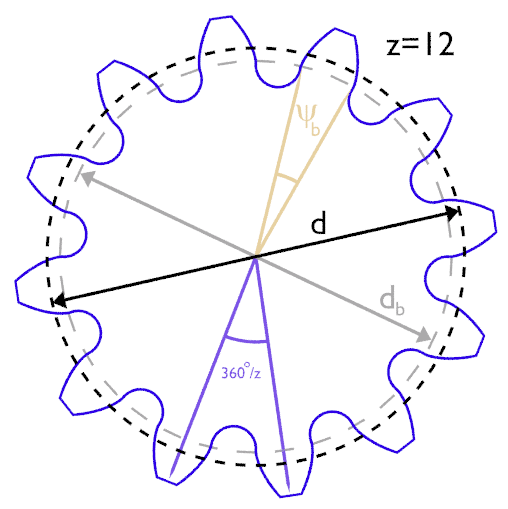Home  •  Tutorials  •  Calculators  •  3D Models  •  About  •  Contact

Involute Gear Calculator

The following online calculator computes the basic dimensions and tooth profile of an involute gear based on its module, number of teeth and pressure angle (the latter is usually 20°).A gear wheel can be fully defined with as few as two parameters: the number of teeth (z) and module (m). The latter is simply the reference diameter divided by the number of teeth. Changing the other two parameters (α and x) is not recommended.

Basic Dimensions
ItemSymbolValue
Module m
Number of teethz
Pressure Angle (°) α
Profile shiftx
Reference diameterd
Base diameterdb